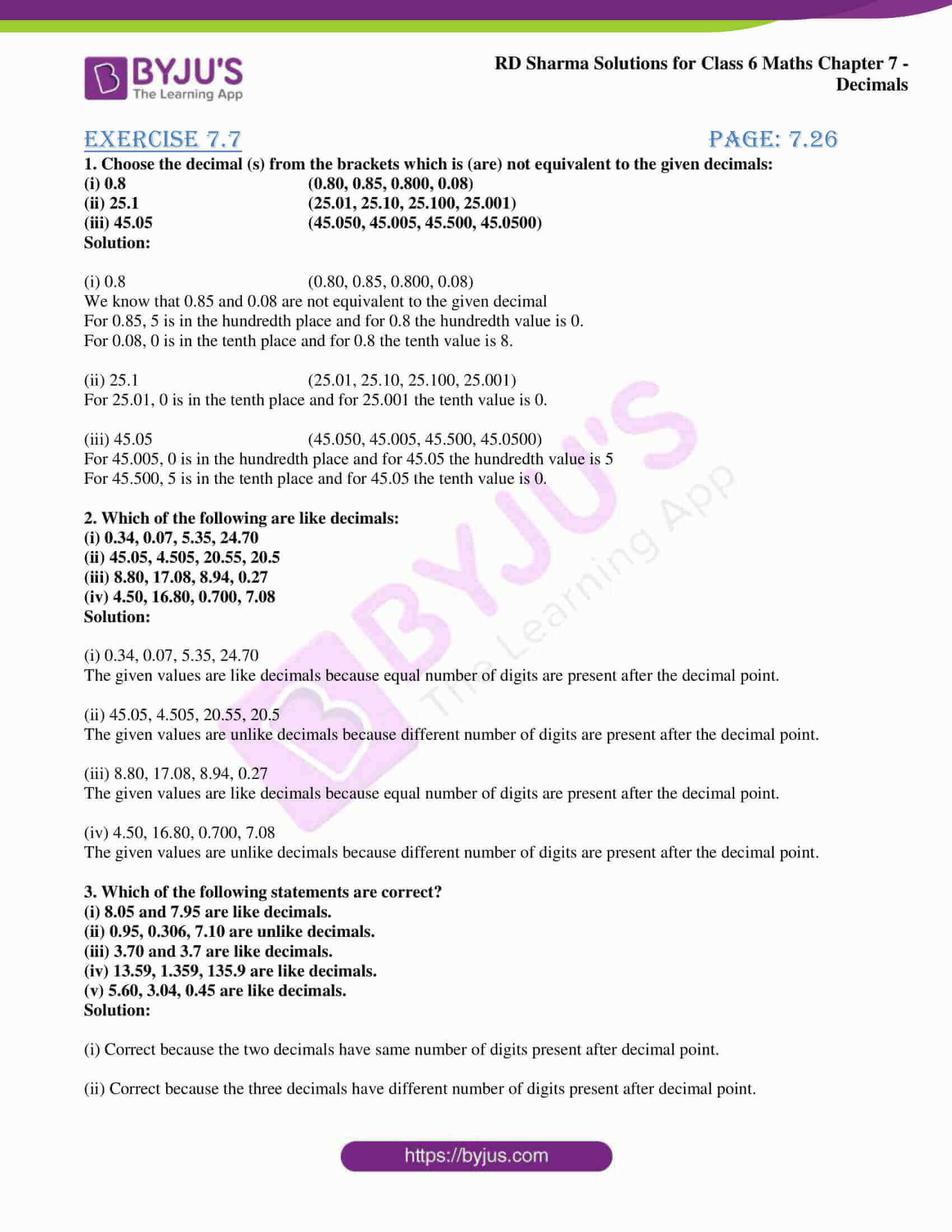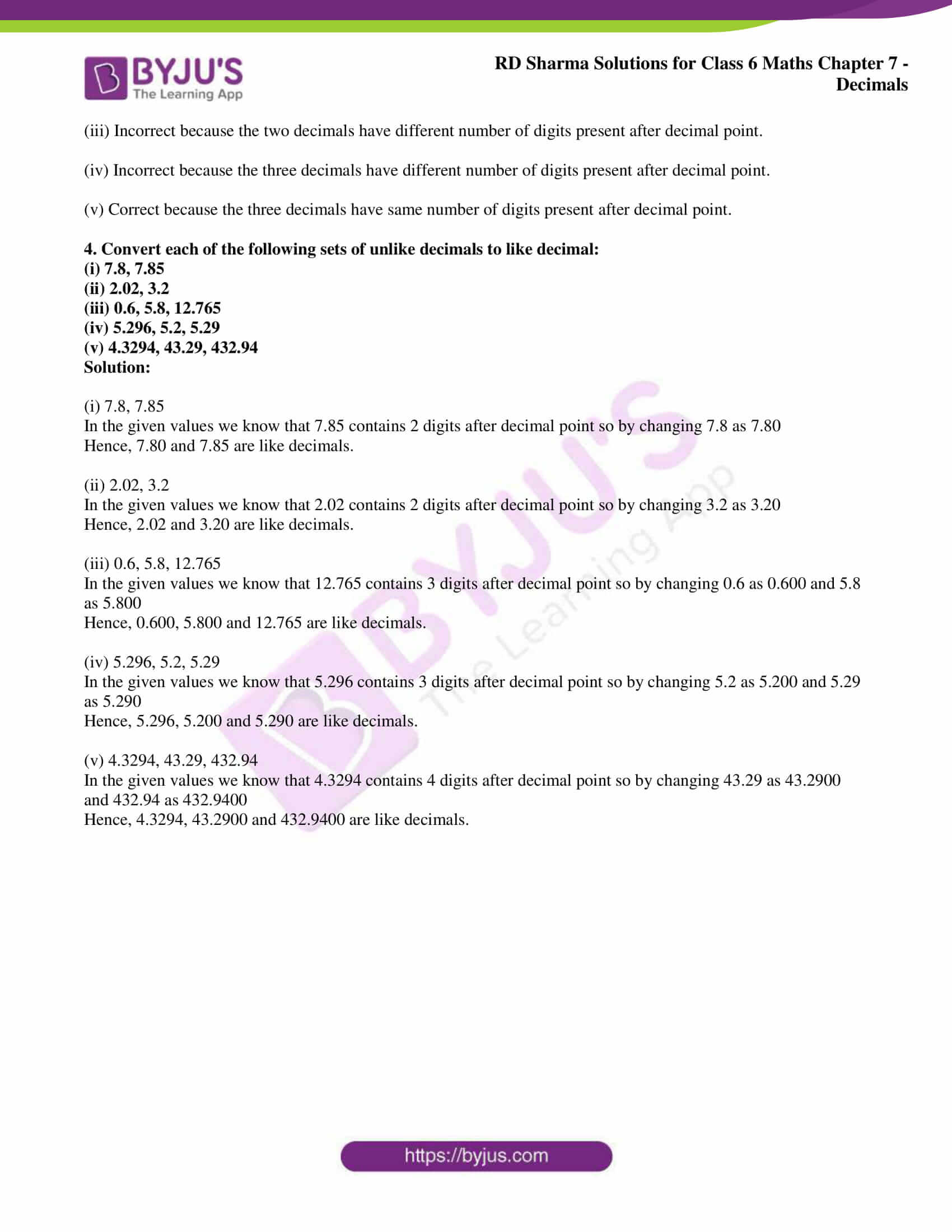# RD Sharma Solutions for Class 6 Chapter 7: Decimals Exercise 7.7

Exercise 7.7 of RD Sharma Solutions Class 6 explains important concepts like equivalent decimals, like and unlike decimals and conversion of unlike decimals to like decimals. Numbers which are obtained by inserting zeros after the extreme right digit in the decimal part of a decimal number are called equivalent decimals. Numbers which have the same number of digits after the decimal point are like decimals, and that which have different digits are unlike decimals. RD Sharma Solutions for Class 6 Maths Chapter 7 Decimals Exercise 7.7 PDF which are provided here.

## RD Sharma Solutions for Class 6 Chapter 7: Decimals Exercise 7.7 Download PDF### Access RD Sharma Solutions for Class 6 Chapter 7: Decimals Exercise 7.7

1. Choose the decimal (s) from the brackets which is (are) not equivalent to the given decimals:

(i) 0.8 (0.80, 0.85, 0.800, 0.08)

(ii) 25.1 (25.01, 25.10, 25.100, 25.001)

(iii) 45.05 (45.050, 45.005, 45.500, 45.0500)

Solution:

(i) 0.8 (0.80, 0.85, 0.800, 0.08)

We know that 0.85 and 0.08 are not equivalent to the given decimal

For 0.85, 5 is in the hundredth place and for 0.8 the hundredth value is 0.

For 0.08, 0 is in the tenth place and for 0.8 the tenth value is 8.

(ii) 25.1 (25.01, 25.10, 25.100, 25.001)

For 25.01, 0 is in the tenth place and for 25.001 the tenth value is 0.

(iii) 45.05 (45.050, 45.005, 45.500, 45.0500)

For 45.005, 0 is in the hundredth place and for 45.05 the hundredth value is 5

For 45.500, 5 is in the tenth place and for 45.05 the tenth value is 0.

2. Which of the following are like decimals:

(i) 0.34, 0.07, 5.35, 24.70

(ii) 45.05, 4.505, 20.55, 20.5

(iii) 8.80, 17.08, 8.94, 0.27

(iv) 4.50, 16.80, 0.700, 7.08

Solution:

(i) 0.34, 0.07, 5.35, 24.70

The given values are like decimals because equal number of digits are present after the decimal point.

(ii) 45.05, 4.505, 20.55, 20.5

The given values are unlike decimals because different number of digits are present after the decimal point.

(iii) 8.80, 17.08, 8.94, 0.27

The given values are like decimals because equal number of digits are present after the decimal point.

(iv) 4.50, 16.80, 0.700, 7.08

The given values are unlike decimals because different number of digits are present after the decimal point.

3. Which of the following statements are correct?

(i) 8.05 and 7.95 are like decimals.

(ii) 0.95, 0.306, 7.10 are unlike decimals.

(iii) 3.70 and 3.7 are like decimals.

(iv) 13.59, 1.359, 135.9 are like decimals.

(v) 5.60, 3.04, 0.45 are like decimals.

Solution:

(i) Correct because the two decimals have same number of digits present after decimal point.

(ii) Correct because the three decimals have different number of digits present after decimal point.

(iii) Incorrect because the two decimals have different number of digits present after decimal point.

(iv) Incorrect because the three decimals have different number of digits present after decimal point.

(v) Correct because the three decimals have same number of digits present after decimal point.

4. Convert each of the following sets of unlike decimals to like decimal:

(i) 7.8, 7.85

(ii) 2.02, 3.2

(iii) 0.6, 5.8, 12.765

(iv) 5.296, 5.2, 5.29

(v) 4.3294, 43.29, 432.94

Solution:

(i) 7.8, 7.85

In the given values we know that 7.85 contains 2 digits after decimal point so by changing 7.8 as 7.80

Hence, 7.80 and 7.85 are like decimals.

(ii) 2.02, 3.2

In the given values we know that 2.02 contains 2 digits after decimal point so by changing 3.2 as 3.20

Hence, 2.02 and 3.20 are like decimals.

(iii) 0.6, 5.8, 12.765

In the given values we know that 12.765 contains 3 digits after decimal point so by changing 0.6 as 0.600 and 5.8 as 5.800

Hence, 0.600, 5.800 and 12.765 are like decimals.

(iv) 5.296, 5.2, 5.29

In the given values we know that 5.296 contains 3 digits after decimal point so by changing 5.2 as 5.200 and 5.29 as 5.290

Hence, 5.296, 5.200 and 5.290 are like decimals.

(v) 4.3294, 43.29, 432.94

In the given values we know that 4.3294 contains 4 digits after decimal point so by changing 43.29 as 43.2900 and 432.94 as 432.9400

Hence, 4.3294, 43.2900 and 432.9400 are like decimals.